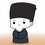# Limit with Power Mean

If $$p$$ is a non-zero real number, and $$x_1,x_2,\dots,x_n$$ are positive real numbers, then the power mean with exponent $$p$$ of these positive real numbers is:

$M_p(x_1,x_2,\dots,x_n)=\left(\frac 1n\sum_{i=1}^n x_i^p\right)^{\frac 1p}.$

For $p=0$ we set it equal to the geometric mean (which is the limit of means with exponents approaching zero):

$M_0(x_1,x_2,\dots,x_n)=\sqrt[n]{\prod_{i=1}^n x_i}.$

Evaluate the limit below for all real number $p$:

$\lim_{n\to\infty}\sqrt[n]{M_p\left(\binom n0,\binom n1,\dots,\binom nn\right)}.$

I got the incomplete answer (see problems Interesting Limit 24, 21 and wiki power mean inequality):

$\lim_{n\to\infty}\sqrt[n]{M_p\left(\binom n0,\binom n1,\dots,\binom nn\right)}= \begin{cases} 1,&\text{if }p\le -1\\ \sqrt e,&\text{if }p=0\\ 2,&\text{if }p\ge 1 \end{cases}$

Using Aaghaz Mahajan's result below, further answer is obtained:

$\lim_{n\to\infty}\sqrt[n]{M_p\left(\binom n0,\binom n1,\dots,\binom nn\right)}= \begin{cases} 1,&\text{if }p\le -1\\ \sqrt e,&\text{if }p=0\\ 2,&\text{if }p> 0 \end{cases}$Note by Brian Lie
2 years, 2 months ago

This discussion board is a place to discuss our Daily Challenges and the math and science related to those challenges. Explanations are more than just a solution — they should explain the steps and thinking strategies that you used to obtain the solution. Comments should further the discussion of math and science.

When posting on Brilliant:

• Use the emojis to react to an explanation, whether you're congratulating a job well done , or just really confused .
• Ask specific questions about the challenge or the steps in somebody's explanation. Well-posed questions can add a lot to the discussion, but posting "I don't understand!" doesn't help anyone.
• Try to contribute something new to the discussion, whether it is an extension, generalization or other idea related to the challenge.

MarkdownAppears as
*italics* or _italics_ italics
**bold** or __bold__ bold
- bulleted- list
• bulleted
• list
1. numbered2. list
1. numbered
2. list
Note: you must add a full line of space before and after lists for them to show up correctly
paragraph 1paragraph 2

paragraph 1

paragraph 2

[example link](https://brilliant.org)example link
> This is a quote
This is a quote
    # I indented these lines
# 4 spaces, and now they show
# up as a code block.

print "hello world"
# I indented these lines
# 4 spaces, and now they show
# up as a code block.

print "hello world"
MathAppears as
Remember to wrap math in $$ ... $$ or $ ... $ to ensure proper formatting.
2 \times 3 $2 \times 3$
2^{34} $2^{34}$
a_{i-1} $a_{i-1}$
\frac{2}{3} $\frac{2}{3}$
\sqrt{2} $\sqrt{2}$
\sum_{i=1}^3 $\sum_{i=1}^3$
\sin \theta $\sin \theta$
\boxed{123} $\boxed{123}$

Sort by:

@Brian Lie Sir, could you suggest me any books to learn Dynamics from?? Or Physics in general??

- 2 years, 2 months ago

May I say something? I asked same question to Josh Silverman 3 years ago, he answered Kleppner and Klowenkow is the best.

- 2 years, 2 months ago

- 2 years, 2 months ago

Mechanics and Special Relativity by Freddie Williams is more mathematical.

- 2 years, 2 months ago

Thanks Sir....!!

- 2 years, 2 months ago

@Brian Lie Hello!! I think this result might help you for positive 'p'...........I have read it in a book, I can send you the book if you want.......

- 2 years, 2 months ago

Dear Aaghaz, my email address is lixuepeili@gmail.com. Thank you for your sending.

- 2 years, 2 months ago